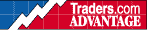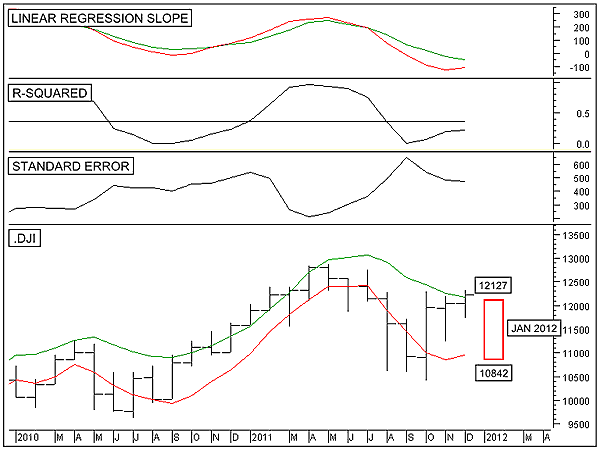HOT TOPICS LIST

INDICATORS LIST

LIST OF TOPICS

# As January 2012 Goes

01/04/12 12:08:27 PM
by Alan R. Northam

As January goes, so goes the year. Here, I use statistical analysis to forecast what the stock market will do in January and the rest of 2012.

Security:   .DJI
Position:   N/A

 The January barometer, devised by Yale Hirsch in 1972, states that as January goes, so goes the year. This barometer of the stock market has only been wrong six times since 1950 for a 90% accuracy ratio. At the beginning of 2011, I wrote an article entitled "As January Goes." This article made a prediction as to whether the Dow Jones Industrial Average (DJIA) would show a gain or a loss for the year. This prediction was based upon Hirsch's work and statistical analysis. The prediction stated that January would be a flat month and the DJIA would end the year close to where it started. As the prediction stated, 2011 did turn out to be relatively flat, with the DJIA ending the year near where it started with a mere 5.5% gain. With 2011 behind us, it is now time to make a new prediction for 2012. Figure 1 shows the monthly time frame of the DJIA. To start the prediction, I first look for a time frame in which the standard error indicator is low and pointing lower. Low standard errors extrapolate to more accurate predictions. By plotting the standard error indicator and changing its time period from 10 upward, I found that 11 periods gave the lowest standard error, with the slope of the error pointing lower. This translates into the smallest deviations in price around the linear regression line and thus the smallest error in predictions.Next, I want to check the strength of the trend. To do this, I plot an R-squared indicator just above the standard error indicator, setting its time frame to 11. This indicator reading is below its critical level, indicating a weak trend. The higher the R-squared value, the more closely price is following the linear regression line and the more accurate the prediction.After that, I want to measure the slope of the linear regression line. To do this, I plot the linear regression slope indicator. Here, I actually plot two linear regression slope indicators. The green indicator represents the slope of the linear regression line of the highest prices of the DJIA for each month over the past 11 months. The value of this slope at the right edge of the chart is -49 points. This means that each month, the linear regression line moves downward at the rate of 49 points, indicating a downtrend. The red line represents the slope of the linear regression line of the lowest prices for each month over the past 11 months. The value of the slope of this linear regression line at the right edge of the chart is -108 points, indicating that the linear regression line is moving downward at the rate of 108 points per month. I use these high and low linear regression line slope measurements to determine the high and low price range over the following month, such as January. This same process can be used each month to predict the next month's price range.FIGURE 1: .DJI, MONTHLY. This chart shows the monthly price chart of the DJIA in the bottom panel, the linear regression slope indicator in the top panel, the R-squared indicator in the second panel, and the standard error indicator in the third. Graphic provided by: MetaStock. Next, I plot two linear regression lines on the price chart. The green line represents the linear regression line of the high prices over the past 11 months. The value of the green linear regression line at the right edge of the chart is 12176. To plot where the high price will be one month into the future, I simply subtract 49 points from 12176 to arrive at a high price of 12127 for January 2012. The red line represents the linear regression line of the low prices over the last 11 months. The value of the red linear regression line at the right edge of the chart is 10950. To plot where the low price for January 2012, I simply subtract 108 from 10950 to arrive at a low price of 10842. These high and low price ranges for January 2012 are then plotted on the chart, as shown by the red rectangle. In conclusion, this prediction gives the high and low price range expectation of the DJIA for January 2012. From this prediction we can expect that January will be a down month, which in turn indicates that the DJIA will end the year lower than where it started. However, a close above the expected price range for January would negate this prediction and would instead forecast higher prices for 2012. A breakdown below the low price prediction for January would also predict lower prices for the year. Having made this forecast, be aware that this is not a strong forecast, as the R-squared indicator is currently below its critical level, indicating a weak downtrend. This raises the possibility that the forecast for January could be wrong and the month could end higher instead of lower. However, once January is over, we should know, with a 90% accuracy, what the prediction is for the rest of 2012. Note: Predictions are just that, predictions. It is a prediction as to what the stock market is expected to do in the future and not what it will do. Good trading to all during 2012.

Alan R. Northam

Alan Northam lives in the Dallas, Texas area and as an electronic engineer gave him an analytical mind from which he has developed a thorough knowledge of stock market technical analysis. His abilities to analyze the future direction of the stock market has allowed him to successfully trade of his own portfolio over the last 30 years. Mr. Northam is now retired and trading the stock market full time. You can reach him at inquiry@tradersclassroom.com or by visiting his website at http://www.tradersclassroom.com. You can also follow him on Twitter @TradersClassrm.

 Comments or Questions? Article Usefulness 5 (most useful) 4 3 2 1 (least useful)